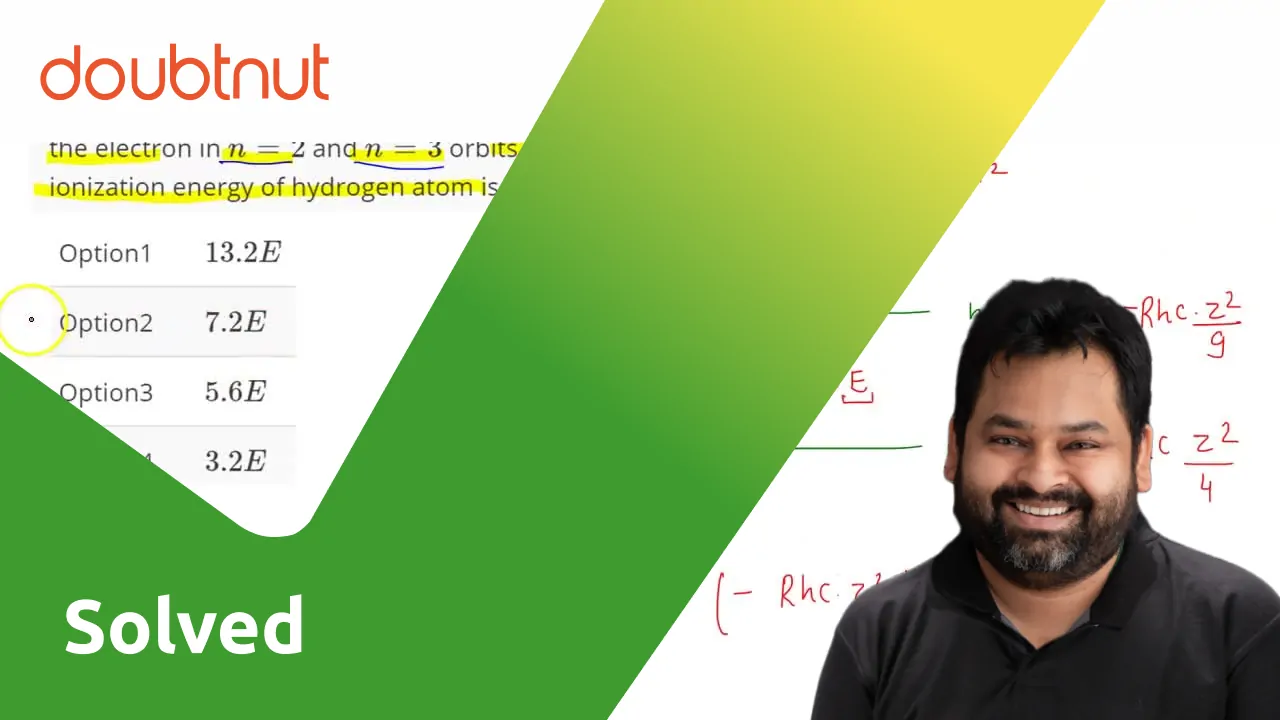Doubtnut App - Get 50 Lakh+ video solutions better than GoogleInstall Now

# In hydrogen atom, if the difference in the energy of the electron in n=2 and n=3 orbits is E, the ionization energy of hydrogen atom is

A

13.2E

B

7.2E

C

5.6E

D

3.2E

Text Solution
Verified by Experts

## Energy E=K[1n21−1n22] (K= constant) n1=2 and n2=3, so E=K[122−132]=K For removing an electro n1=1 to n2=∞ Energy E1=K365E=7.2E ∴ Ionization energy =7.2E